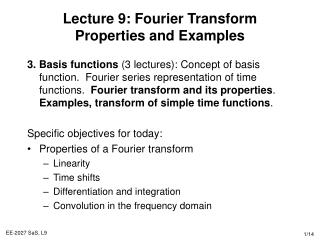DownloadDownload PresentationLecture 9: Fourier Transform Properties and Examples

Lecture 9: Fourier Transform Properties and Examples

Download PresentationLecture 9: Fourier Transform Properties and Examples

- - - - - - - - - - - - - - - - - - - - - - - - - - - E N D - - - - - - - - - - - - - - - - - - - - - - - - - - -
Presentation Transcript

1. Lecture 9: Fourier Transform Properties and Examples • 3. Basis functions (3 lectures): Concept of basis function. Fourier series representation of time functions. Fourier transform and its properties. Examples, transform of simple time functions. • Specific objectives for today: • Properties of a Fourier transform • Linearity • Time shifts • Differentiation and integration • Convolution in the frequency domain

2. Lecture 9: Resources • Core material • SaS, O&W, C4.3, C4.4 • Background material • MIT Lectures 8 and 9.

3. Reminder: Fourier Transform • A signal x(t) and its Fourier transform X(jw) are related by • This is denoted by: • For example (1): • Remember that the Fourier transform is a density function, you must integrate it, rather than summing up the discrete Fourier series components

4. Linearity of the Fourier Transform • If • and • Then • This follows directly from the definition of the Fourier transform (as the integral operator is linear). It is easily extended to a linear combination of an arbitrary number of signals

5. Time Shifting • If • Then • Proof • Now replacing t by t-t0 • Recognising this as • A signal which is shifted in time does not have its Fourier transform magnitude altered, only a shift in phase.

6. Example: Linearity & Time Shift • Consider the signal (linear sum of two time shifted steps) • where x1(t) is of width 1, x2(t) is of width 3, centred on zero. • Using the rectangular pulse example • Then using the linearity and time shift Fourier transform properties

7. Differentiation & Integration • By differentiating both sides of the Fourier transform synthesis equation: • Therefore: • This is important, because it replaces differentiation in the time domain with multiplication in the frequency domain. • Integration is similar: • The impulse term represents the dc or average value that can result from integration

8. Example: Fourier Transform of a Step Signal • Lets calculate the Fourier transform X(jw)of x(t) = u(t), making use of the knowledge that: • and noting that: • Taking Fourier transform of both sides • using the integration property. Since G(jw) = 1: • We can also apply the differentiation property in reverse

9. Convolution in the Frequency Domain • With a bit of work (next slide) it can show that: • Therefore, to apply convolution in the frequency domain, we just have to multiply the two functions. • To solve for the differential/convolution equation using Fourier transforms: • Calculate Fourier transforms of x(t) and h(t) • Multiply H(jw) by X(jw) to obtain Y(jw) • Calculate the inverse Fourier transform of Y(jw) • Multiplication in the frequency domain corresponds to convolution in the time domain and vice versa.

10. Proof of Convolution Property • Taking Fourier transforms gives: • Interchanging the order of integration, we have • By the time shift property, the bracketed term is e-jwtH(jw), so

11. Example 1: Solving an ODE • Consider the LTI system time impulse response • to the input signal • Transforming these signals into the frequency domain • and the frequency response is • to convert this to the time domain, express as partial fractions: • Therefore, the time domain response is: ba

12. H(jw) -wc wc w Example 2: Designing a Low Pass Filter • Lets design a low pass filter: • The impulse response of this filter is the inverse Fourier transform • which is an ideal low pass filter • Non-causal (how to build) • The time-domain oscillations may be undesirable • How to approximate the frequency selection characteristics? • Consider the system with impulse response: • Causal and non-oscillatory time domain response and performs a degree of low pass filtering

13. Lecture 9: Summary • The Fourier transform is widely used for designing filters. You can design systems with reject high frequency noise and just retain the low frequency components. This is natural to describe in the frequency domain. • Important properties of the Fourier transform are: • 1. Linearity and time shifts • 2. Differentiation • 3. Convolution • Some operations are simplified in the frequency domain, but there are a number of signals for which the Fourier transform do not exist – this leads naturally onto Laplace transforms

14. Lecture 9: Exercises • Theory • SaS, O&W, • Q4.6 • Q4.13 • Q3.20, 4.20 • Q4.26 • Q4.31 • Q4.32 • Q4.33